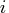Previous Page | Next Page

 Language Reference

## UNIQUEBY Function

returns the locations of the unique by-group combinations for a sorted or indexed matrix

UNIQUEBY( matrix, by, index)

The inputs to the UNIQUEBY function are as follows:

matrix
is the input matrix, which must be sorted or indexed according to the by columns.
by
is either a numeric matrix of column numbers, or a character matrix containing the names of columns corresponding to column labels assigned to matrix by a MATTRIB statement or READ statement.
index
is a vector such that index[] is the row index of theth element of matrix when sorted according to by. Consequently, matrix[index, ] is the sorted matrix. index can be computed for a matrix and a given set of by columns with the SORTNDX call. If the matrix is known to be sorted according to the by columns already, then index should be just 1:nrow(matrix)

The UNIQUEBY function returns a column vector, whoseth row gives the row in index whose value is the row in matrix of theth unique combination of values in the by columns. Suppose you submit the following statement:

```
unique_rows = uniqueby( matrix, by, index);
```

Once you have submitted this statement, the following statement gives the values of the unique by combinations:

```
unique = matrix[ index[ unique_rows ], by ];
```

In addition, the following statement gives the number of unique values:

```
n = nrow( unique );
```

The following statement gives the number of rows in theth by combination, except for the last combination.

```
size = unique_rows[i+1] - unique_rows[i];
```

The last combination is given by the following statement:

```
size_last = nrow(matrix) - unique_rows[nrow(unique_rows)] + 1;
```

If matrix is already sorted according to the by columns (see the SORT call), then UNIQUEBY can be called with 1:nrow(matrix) for the index argument, as follows:

```
unique_loc = uniqueby( matrix, by, 1:nrow(matrix) );
```Previous Page | Next Page | Top of Page